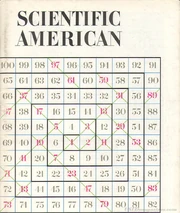## FANDOM

852 Pages

The Ulam spirals are a method of visualising the appearance of primes using spirals.

## ConstructionUlam's spiral The Ulam spirals were originally constructed in 1963 by Stanislaw Ulam, who wrote integers in a spiral starting with one at the centre. He then circled all the primes and noticed that the circled numbers tended to line up on diagonal lines. This was a major discovery in mathematics at the time. These diagonal lines appeared no matter what number was at the centre, showing that these lines are prominent and their appearances are not just coincidental.

## Hardy and Littlewood's Conjecture

One of the diagonal lines in the Ulam spiral follow an equation- $4x^2-2x+1$. A large amount of prime numbers fall on this line. This is a method of finding large primes that is an alternative to Mersenne primes. More importantly, Hardy's conjecture states that there are some equations of the form $ax^2+bx+c$ where the HCF of a, b and c is 1 and where $b^2-4ac$ is not a perfect square with more primes than others. It is conjectured that there is a formula to find these equations.

Community content is available under CC-BY-SA unless otherwise noted.